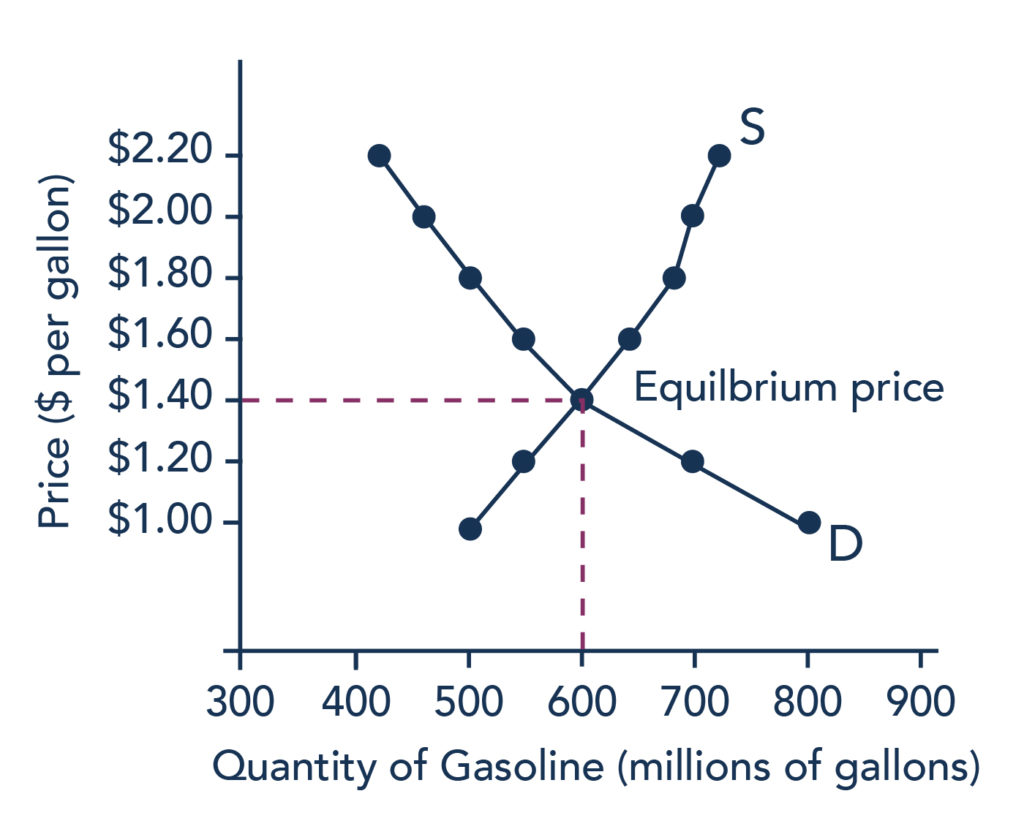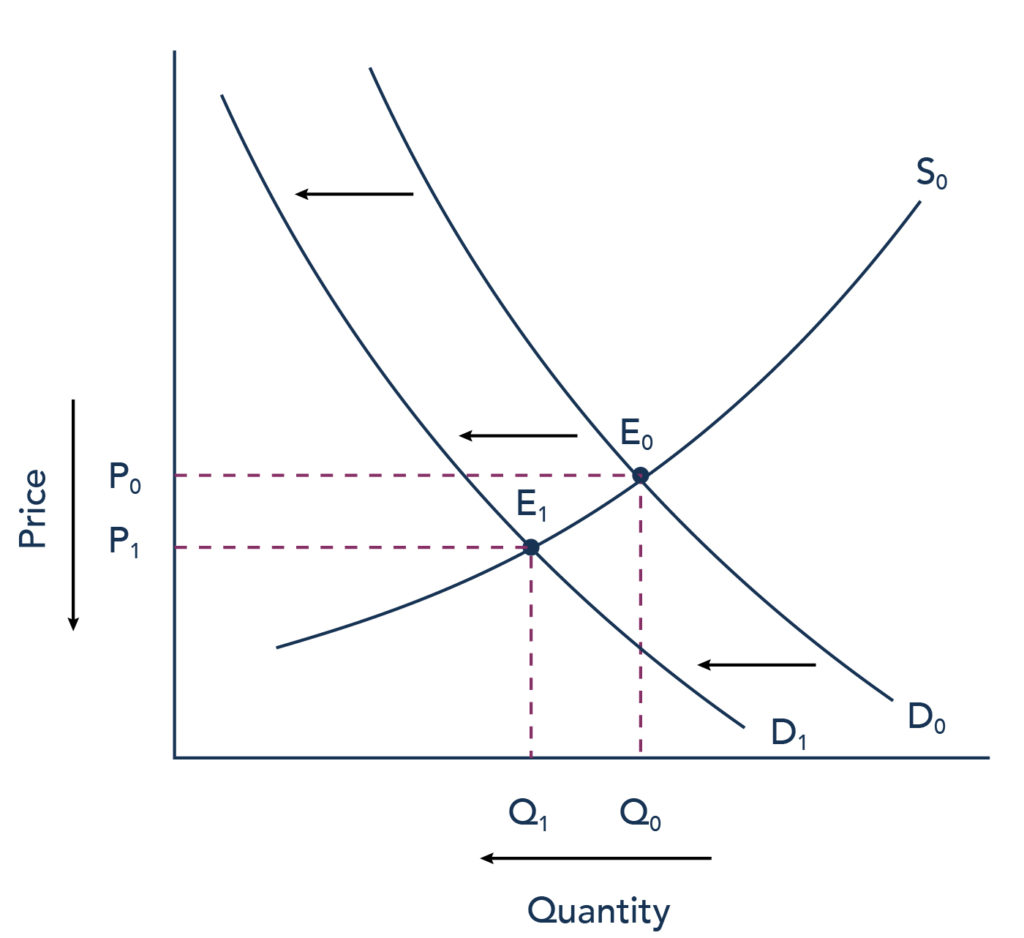## Equilibrium, Price, and Quantity

### Learning Outcomes

• Explain equilibrium price and quantity

## Equilibrium: Where Supply and Demand Intersect

When two lines on a diagram cross, this intersection usually means something. On a graph, the point where the supply curve (S) and the demand curve (D) intersect is the equilibrium. The equilibrium price is the only price where the desires of consumers and the desires of producers agree—that is, where the amount of the product that consumers want to buy (quantity demanded) is equal to the amount producers want to sell (quantity supplied). This mutually desired amount is called the equilibrium quantity. At any other price, the quantity demanded does not equal the quantity supplied, so the market is not in equilibrium at that price. It should be clear, from the previous discussions of surpluses and shortages, that if a  market is not in equilibrium, then market forces will push the market to the equilibrium.

If you have only the demand and supply schedules, and no graph, then you can find the equilibrium by looking for the price level on the tables where the quantity demanded and the quantity supplied are equal (see the numbers in bold in Table 1 in the previous page that indicates this point).

### Finding Equilibrium with Algebra

We’ve just explained two ways of finding a market equilibrium: by looking at a table showing the quantity demanded and supplied at different prices, and by looking at a graph of demand and supply. We can also identify the equilibrium with a little algebra if we have equations for the supply and demand curves. Let’s practice solving a few equations that you will see later in the course. Right now, we are only going to focus on the math. Later, you’ll learn why these models work the way they do, but let’s start by focusing on solving the equations. Suppose that the demand for soda is given by the following equation:

$Qd=16–2P$

where Qd is the amount of soda that consumers want to buy (i.e., quantity demanded), and P is the price of soda. Suppose the supply of soda is

$Qs=2+5P$

where Qs is the amount of soda that producers will supply (i.e., quantity supplied). (Remember, these are simple equations for lines). Finally, recall that the soda market converges to the point where supply equals demand, or

$Qd=Qs$

We now have a system of three equations and three unknowns (Qd, Qs, and P), which we can solve with algebra. Since

$Qd=Qs$,

we can set the demand and supply equations equal to each other:

$\begin{array}{c}\,\,Qd=Qs\\16-2P=2+5P\end{array}$

Step 1: Isolate the variable by adding 2P to both sides of the equation and subtracting 2 from both sides.

$\begin{array}{l}\,16-2P=2+5P\\-2+2P=-2+2P\\\,\,\,\,\,\,\,\,\,\,\,\,\,\,\,14=7P\end{array}$

Step 2: Simplify the equation by dividing both sides by 7.

$\begin{array}{ccc}\dfrac{14}{7}&=&\dfrac{7P}{7}\\2&=&P\end{array}$

The equilibrium price of soda, that is, the price where Qs = Qd, will be $2. Now we want to determine the quantity amount of soda. We can do this by plugging the equilibrium price into either the equation showing the demand for soda or the equation showing the supply of soda. Let’s use demand. Remember, the formula for quantity demanded is the following: $Qd=16-2P$ Taking the price of$2, and plugging it into the demand equation, we get

$\begin{array}{l}Qd=16–2(2)\\Qd=16–4\\Qd=12\end{array}$

So, if the price is $2 each, consumers will purchase 12. How much will producers supply, or what is the quantity supplied? Taking the price of$2, and plugging it into the equation for quantity supplied, we get the following:

$\begin{array}{l}Qs=2+5P\\Qs=2+5(2)\\Qs=2+10\\Qs=12\end{array}$

Now, if the price is $2 each, producers will supply 12 sodas. This means that we did our math correctly, since $Qd=Qs$ and both Qd and Qs are equal to 12. That confirms that we’ve found the equilibrium quantity. ### Watch It Watch this video for a closer look at market equilibrium: Equilibrium occurs at the point where quantity supplied = quantity demanded. ## Equilibrium and Economic Efficiency Equilibrium is important to create both a balanced market and an efficient market. If a market is at its equilibrium price and quantity, then it has no reason to move away from that point, because it’s balancing the quantity supplied and the quantity demanded. However, if a market is not at equilibrium, then economic pressures arise to move the market toward the equilibrium price and equilibrium quantity. This happens either because there is more supply than what the market is demanding, or because there is more demand than the market is supplying. This balance is a natural function of a free-market economy. Also, a competitive market that is operating at equilibrium is an efficient market. Economists typically define efficiency in this way: when it is impossible to improve the situation of one party without imposing a cost on another. Conversely, if a situation is inefficient, then it becomes possible to benefit at least one party without imposing costs on others.Figure 1. Demand and Supply for Gasoline: Equilibrium. At this equilibrium point, the market is efficient because the optimal amount of gasoline is being produced and consumed. Efficiency in the demand and supply model has the same basic meaning: the economy is getting as much benefit as possible from its scarce resources, and all the possible gains from trade have been achieved. In other words, the optimal amount of each good and service is being produced and consumed. ### Practice Questions ## Finding Equilibrium Using the Four-Step Process We know that equilibrium is the place where the supply and demand curves intersect, or the point where buyers want to buy the same amount that sellers want to sell. Let’s take a closer look at how to find the equilibrium point using the four-step process. These steps explain how to first draw the demand and supply curves on a graph and find the equilibrium. Next, consider how an economic change (e.g., a natural disaster, a change in production technology, a change in tastes and preferences, income, etc.) might affect supply or demand, then make adjustments to the graph to identify the new equilibrium point. Step 1. Draw demand and supply curves showing the market before the economic change took place. Think about the shift variables for demand and the shift variables for supply. Using this diagram, find the initial equilibrium values for price and quantity. Step 2. Decide whether the economic change being analyzed affects demand or supply. In other words, does the event refer to something in the list of demand shift variables or supply shift variables? Step 3. Determine whether the effect on demand or supply causes the curve to shift to the right or to the left, and sketch the new demand or supply curve on the diagram. In other words, does the event increase or decrease the amount consumers want to buy or the amount producers want to sell? Step 4. Identify the new equilibrium, and then compare the original equilibrium price and quantity to the new equilibrium price and quantity. Let’s consider one example that involves a shift in supply and one that involves a shift in demand. Then we will consider an example where both supply and demand shift. ### Exercise 1: Good Weather for Salmon Fishing Let’s suppose that during the summer of 2015, weather conditions were excellent for commercial salmon fishing off the California coast. Heavy rains meant higher than normal levels of water in the rivers, which helps the salmon to breed. Slightly cooler ocean temperatures stimulated the growth of plankton, the microscopic organisms at the bottom of the ocean food chain, providing everything in the ocean with a hearty food supply. The ocean stayed calm during fishing season, so commercial fishing operations did not lose many days to bad weather. How did these climate conditions affect the quantity and price of salmon? Let’s consider this situation using the four-step process and the data below. Table 1. Salmon Fishing Price per Pound Quantity Supplied in 2014 Quantity Supplied in 2015 Quantity Demanded$2.00 80 400 840
$2.25 120 480 680$2.50 160 550 550
$2.75 200 600 450$3.00 230 640 350
$3.25 250 670 250$3.50 270 700 200

Let’s walk through the four steps together using this example and see how the graph changes. Use the interactive graph below (Figure 2) by clicking on the arrows at the bottom of the activity to navigate through the steps.

Figure 2 (Interactive Graph). Good Weather for Salmon Fishing: The Four-Step Process.

In short, good weather conditions increased supply of the California commercial salmon. The result was a higher equilibrium quantity of salmon bought and sold in the market at a lower price.

### Exercise 2: Newspapers and the Internet

According to the Pew Research Center for People and the Press, more and more people, especially younger people, are getting their news from online and digital sources. The majority of U.S. adults now own smartphones or tablets, and most of those Americans say they use them in part to get the news. From 2004 to 2012, the share of Americans who reported getting their news from digital sources increased from 24 percent to 39 percent. How has this trend affected consumption of print news media and radio and television news? Figure 3 and the text below illustrate the four-step analysis used to answer this question.Figure 3. Graph depicting the changing market for print news.

Step 1. Draw a demand and supply model to think about what the market looked like before the event.

The demand curve D0 and the supply curve S0 show the original relationships. In this case, the curves are drawn without specific numbers on the price and quantity axis.

Step 2. Did the change described affect supply or demand?

Step 3. Was the effect on demand positive or negative?

Step 4. Compare the new equilibrium price and quantity to the original equilibrium price.

The decline in print news reading predates 2004. Print newspaper circulation peaked in 1973 and has declined since then due to competition from television and radio news. Fifty-five percent of Americans in 1991 indicated that they got their news from print sources, while only 29 percent did so in 2012. Radio news has followed a similar path in recent decades, with the share of Americans getting their news from radio declining from 54 percent in 1991 to 33 percent in 2012. Television news has held its own during the last fifteen years, with the market share staying in the mid- to upper-fifties. What does this suggest for the future, given that two-thirds of Americans under 30 years old say they don’t get their news from television at all?

## Try It

Play the simulation below multiple times to see how different choices lead to different outcomes. All simulations allow unlimited attempts, so that you can gain experience applying the concepts.

## Contribute!

Did you have an idea for improving this content? We’d love your input.